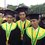# One Real Root

Given a equation:$a^x-x=0$ for $a>1$

Determine value of $a$ so that the equation has exactly 1 real rootNote by Idham Muqoddas
6 years, 9 months ago

This discussion board is a place to discuss our Daily Challenges and the math and science related to those challenges. Explanations are more than just a solution — they should explain the steps and thinking strategies that you used to obtain the solution. Comments should further the discussion of math and science.

When posting on Brilliant:

• Use the emojis to react to an explanation, whether you're congratulating a job well done , or just really confused .
• Ask specific questions about the challenge or the steps in somebody's explanation. Well-posed questions can add a lot to the discussion, but posting "I don't understand!" doesn't help anyone.
• Try to contribute something new to the discussion, whether it is an extension, generalization or other idea related to the challenge.

MarkdownAppears as
*italics* or _italics_ italics
**bold** or __bold__ bold
- bulleted- list
• bulleted
• list
1. numbered2. list
1. numbered
2. list
Note: you must add a full line of space before and after lists for them to show up correctly
paragraph 1paragraph 2

paragraph 1

paragraph 2

[example link](https://brilliant.org)example link
> This is a quote
This is a quote
    # I indented these lines
# 4 spaces, and now they show
# up as a code block.

print "hello world"
# I indented these lines
# 4 spaces, and now they show
# up as a code block.

print "hello world"
MathAppears as
Remember to wrap math in $$ ... $$ or $ ... $ to ensure proper formatting.
2 \times 3 $2 \times 3$
2^{34} $2^{34}$
a_{i-1} $a_{i-1}$
\frac{2}{3} $\frac{2}{3}$
\sqrt{2} $\sqrt{2}$
\sum_{i=1}^3 $\sum_{i=1}^3$
\sin \theta $\sin \theta$
\boxed{123} $\boxed{123}$

Sort by:

Consider two curves $y = a^x$ and $y = x$. Since , the two curves would intersect at only one point , we would better like to say that the two curve would touch each other. Or they have same derivative at the point of contact, taking derivative of both, $a^x lna = 1$ $\Rightarrow$ $a^x = \frac{1}{lna}$ . Since $a^x = x$ $\Rightarrow$ $x = \frac{1}{lna}$. Hence we get that $a^{\frac{1}{lna}} = \frac{1}{lna}$. Now $\frac{1}{lna} = log_{a}e$ . Hence LHS becomes e. $\Rightarrow e = log_{a}e \Rightarrow lna = \frac{1}{e} \Rightarrow a = e^{\frac{1}{e}}$.

- 6 years, 9 months ago

I request someone to tell me how to break the lines in formatting, the above lines look together but weren't written together

- 6 years, 9 months ago

If you want to separate paragraphs, you need to leave at least one clear blank line between them. Press the carriage return twice, not once, at the end of each paragraph!

- 6 years, 9 months ago

thanks!

- 6 years, 9 months ago

thank you so much Jatin

- 6 years, 9 months ago

Now , this method can be extended to a lot of problems .

For example , find the individual values of a so that the given equations have only one solution.

1. $e^x - ax =0$

2. $e^x - ax^2 =0$

3. $x = ln(ax)$ , etc.

- 6 years, 9 months ago

$a = e^{\frac{1}{e}}$

- 6 years, 9 months ago

- 6 years, 9 months ago

Since $a^x > 0$ for all real $x$, we deduce that the only possible solutions of this equation must be positive. Thus we can restrict attention to positive $x$, and then this equation is equivalent to $\frac{\ln x}{x} \; = \; \ln a$ The function $y = \tfrac{\ln x}{x}$ increases from $-\infty$ to $e^{-1}$ as $x$ varies from $0$ to $e$, and decreases from $e^{-1}$ to $0$ as $x$ increases from $e$ to $\infty$. Thus

• The equation has a unique positive solution lying in $(0,1]$ for any $0 < a \le 1$, but we are not interested in this case.

• The equation has two positive real solutions lying in $(1,\infty)$ for any $1 < a < e^{\frac{1}{e}}$.

• The equation has a unique real solution $x=e$ for $a = e^{\frac{1}{e}}$.

• The equation has no real solutions for $a > e^{\frac{1}{e}}$.

- 6 years, 9 months ago

$y = x$ is a line with slope 1 passing through the origin. $y = a^x$ is a curve which contains the point $(0, 1)$ and grows exponentially to the right of this point and approaches $y = 0$ to the left of this point for $a > 1$. If there is only one solution for $x$, then necessarily these two graphs intersect only once, meaning that they have the same derivative at their intersection. Taking derivatives of both we get $a^x * ln a = 1$.

Substituting $a^x = x$ we get $x = \frac {1}{ln a}$. Now, substituting this back in to the original equation we get $a^{\frac {1}{ln a}} = \frac {1}{ln a} = \frac {ln e}{ln a} = log_ae \Rightarrow a^{\frac {1}{ln a}} = e = \frac{1}{ln a} \rightarrow ln a = \frac {1}{e} \Rightarrow a = e^{\frac {1}{e}}$

- 6 years, 9 months ago